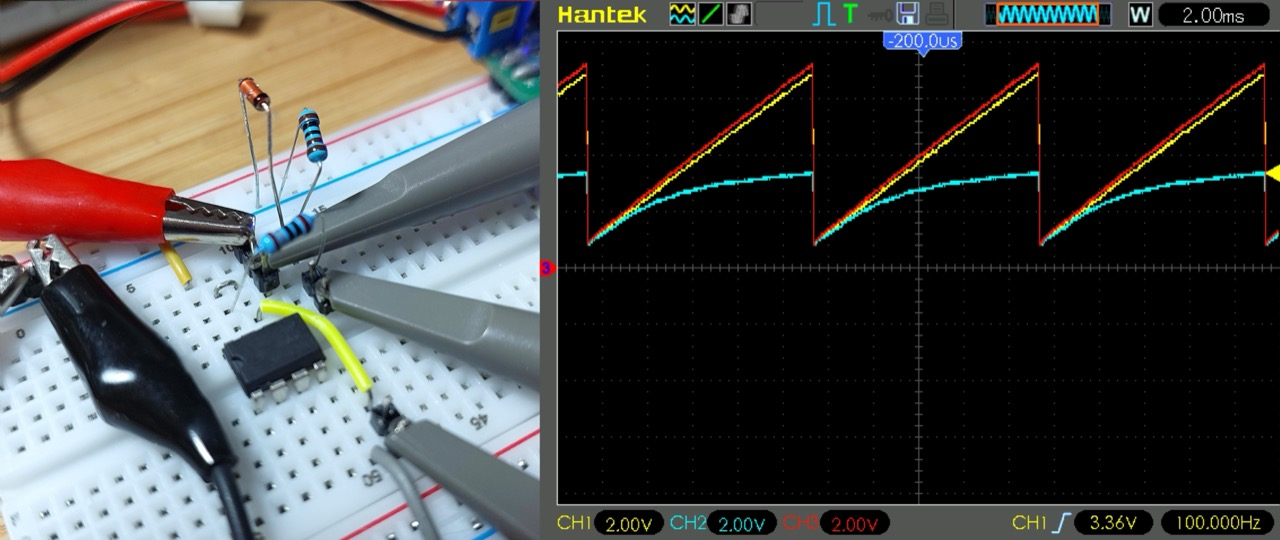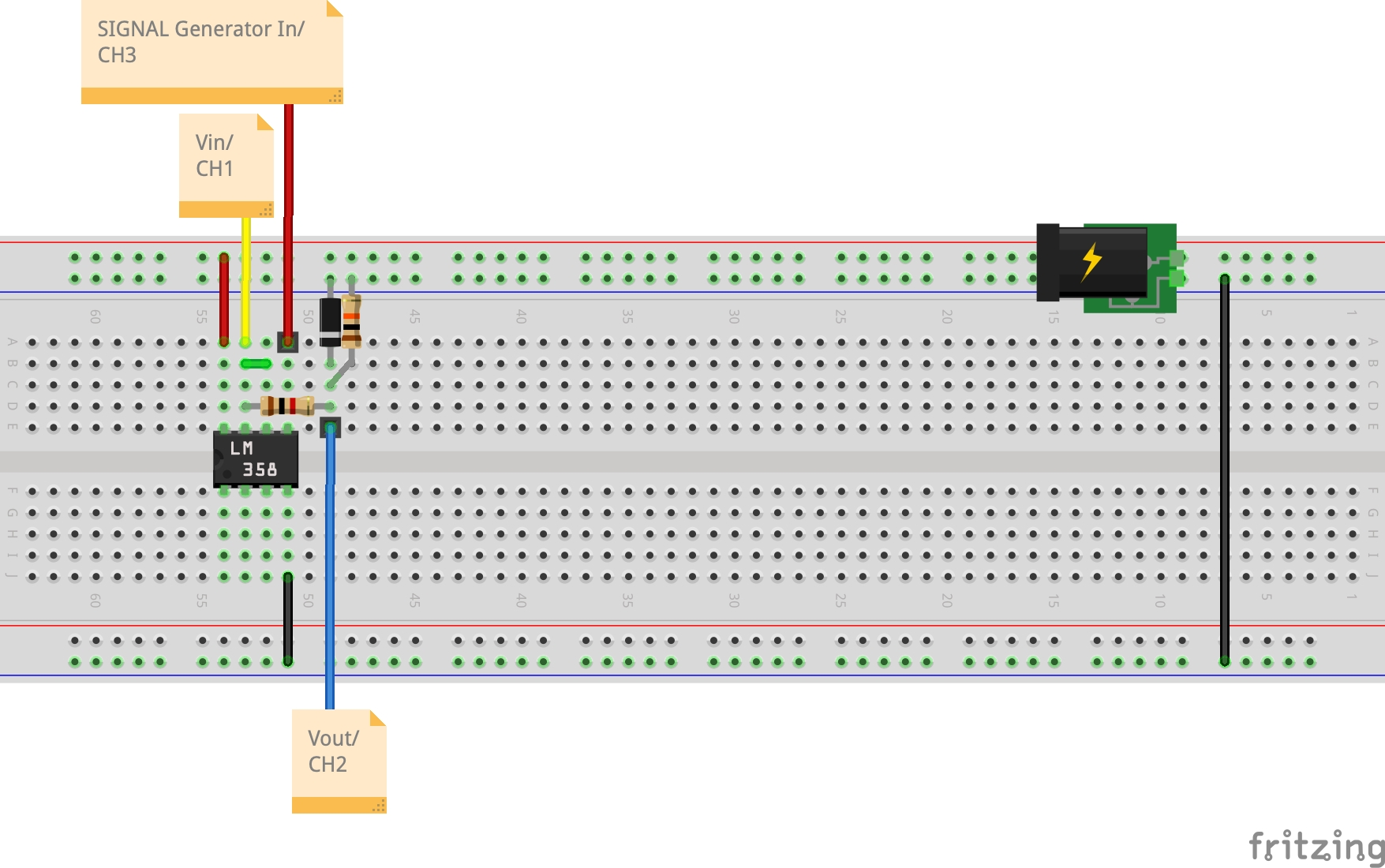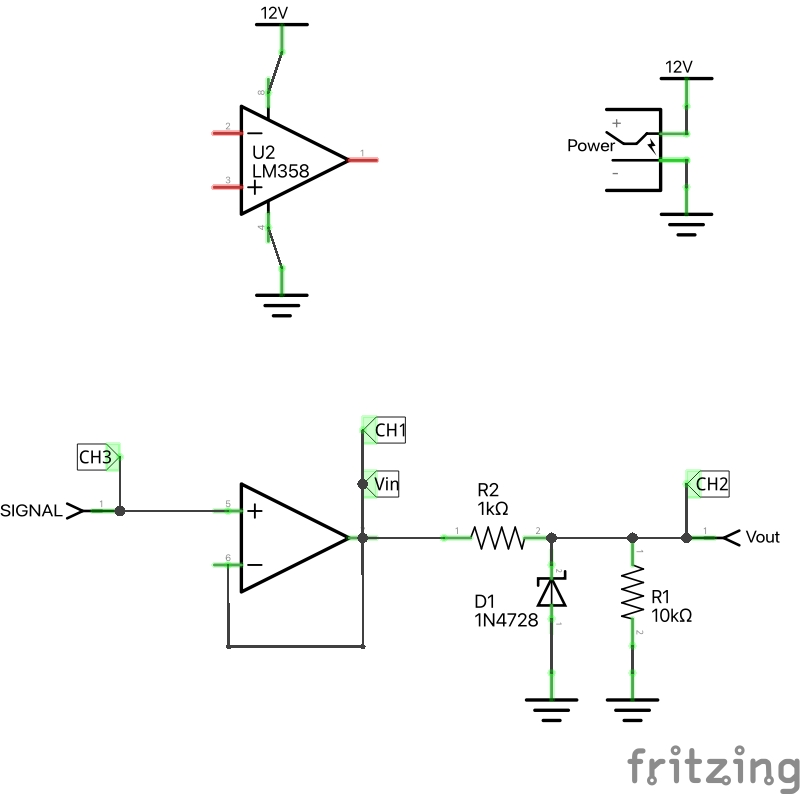# #029 Zener Regulator

Demonstrates using a 1N4733 Zener diode as a 5V regulator, using an Arduino to measure the voltage supply and plot the results with Processing.The 1N4733 zener diode is rated at 5.1V reverse-bias beakdown voltage. In reverse bias mode, it is able to sustain high current at a very constant value of the reverse-breakdown voltage. Note that other diodes in the 1N4728 - 1N4764 range are available for other zener voltages.

For the zener diode under test, we get a stable voltage just a tad over 5V, as measure with a multimeter and the Arduino:

Vout Derivation
5.05 actual (DMM)
5.05 actual (Arduino)

Here’s a sample trace of the voltage as measured by the Arduino:### Measure Voltage with an Arduino

Reading voltages with analog pins presents two challenges:

• The internal ADC has 10 bit resolution (0 - 1023) with 2 bit precision, so the accuracy is 0.25%
• The internal reference voltage (i.e. what 1023 will equal in volts) is nominally 5V but in practice is not very accurate (the board used for this test runs at about 4.93V)

For our purposes here, the ADC accuracy is not a big problem. To overcome the reference voltage accuracy issue, the sketch scales the voltage reading according to the actual reference voltage of the board. This must be measured with a multimeter and the `vref_mv` constant changed to this value (in mV).

For more detail, John Errington’s Experiments with an Arduino includes an excellent study of Precise voltage measurement with the Arduino.

### Construction

The Arduino only acts as a measurement device in this circuit. Analog pin is used to read Vout via a voltage divider (Rd1/Rd2). The voltage divider is to ensure that the Arduino never sees more than half the battery voltage (4.5V) on the analog pin.

PlotNValues (a simple Processing sketch) reads the data from the serial port and plots the input and output value over time, with some coloration effects thrown in for good measure. In other words, we’re using Arduino and Processing as a basic oscilloscope! And it kind of works, mainly because the frequency is so low.